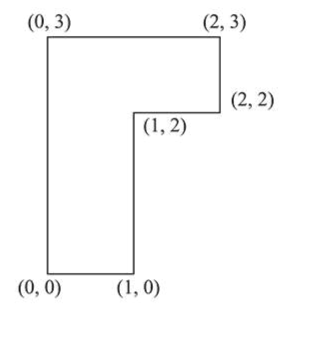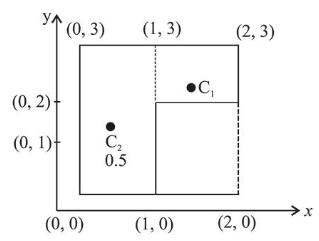# The coordinates of centre of mass of a uniform flag shaped laminaQuestion:

The coordinates of centre of mass of a uniform flag shaped lamina (thin flat plale) of mass $4 \mathrm{~kg}$. (The coordinates of the same are shown in figure) are:1. (1) $(1.25 \mathrm{~m}, 1.50 \mathrm{~m})$

2. (2) $(0.75 \mathrm{~m}, 1.75 \mathrm{~m})$

3. (3) $(0.75 \mathrm{~m}, 0.75 \mathrm{~m})$

4. (4) $(1 \mathrm{~m}, 1.75 \mathrm{~m})$

Correct Option: , 2

Solution:

(2)For given Lamina

$x y$

$(1.5,2.5)$

$m_{1}=1, C_{1}=$

$m_{2}=3, C_{2}=$ $(0.5,1.5)$

$X_{c m}=\frac{m_{1} x_{1}+m_{2} x_{2}}{m_{1}+m_{2}}=\frac{1.5+1.5}{4}=0.75$

$Y_{c m}=\frac{m_{1} y_{1}+m_{2} y_{2}}{m_{1}+m_{2}}=\frac{2.5+4.5}{4}=1.75$

$\therefore$ Coordinate of centre of mass of flag shaped lamina

$(0.75,1.75)$